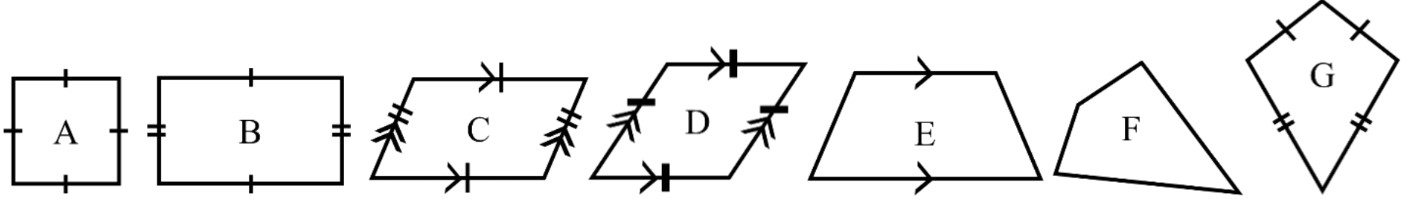# Classifying quadrilaterals

### Classifying quadrilaterals

#### Lessons

In this lesson, we will learn:

• A quadrilateral is a polygon with 4 sides
• We can look at the properties of a quadrilateral (pairs of parallel sides, pairs of congruent sides, types of angles) to classify into 6 different types
• The special types of quadrilaterals are: rectangle, square, parallelogram, rhombus, trapezoid, and kite

Notes:

• A quadrilateral is a 2D shape with 4 straight sides (a 4-sided polygon)
• A quadrilateral also has 4 vertices (corners)
• Each corner contains an angle; the total angle sum in any quadrilateral is 360°

• There are special quadrilaterals with specific definitions and names
• There will be 6 types of quadrilaterals in this lesson: Rectangle, Square, Parallelogram, Rhombus, Trapezoid, and Kite
• A quadrilateral can fit the definition of more than one of these special types

• Rectangles and squares:
• Quadrilaterals with 4 right angles (all angles are 90° )
• A rectangle has 2 pairs of opposite and equal sides (congruent)
• A square is a special type of rectangle where all 4 sides are equal (congruent)
• All squares are rectangles, but not all rectangles are squares
• Rectangles and squares can also be considered as parallelograms, just with right angles

• Parallelograms and rhombuses:
• Quadrilaterals with 2 pairs of parallel sides and 2 pairs of opposite equal angles
• A parallelogram has 2 pairs of congruent sides (equal length)
• A rhombus has all 4 sides congruent (all 4 sides equal length)
• All rhombuses are parallelograms; not all parallelograms are rhombuses

• Trapezoids:
• Quadrilaterals with only 1 pair of parallel sides

• Kites:
• Quadrilaterals with 2 adjacent congruent sides (no parallel sides)
• There is 1 pair of opposite equal angles across the line of symmetry

• Summary of quadrilateral types and properties (sides and angles):• Introduction
Introduction to Classifying Quadrilaterals:
a)
What is a quadrilateral and what geometry concepts are we going to use to classify different types?

b)
Classifying rectangles and squares

c)
Classifying parallelograms and rhombuses

d)
Classifying trapezoids

e)
Classifying kites

f)
A review on the 6 different classifications of quadrilaterals

• 1.
Naming quadrilateral shapes
Classify the quadrilateral. Think about its sides and angles.
a)b)c)d)e)f)• 2.
Classifying quadrilateral types
Answer with the letter on each quadrilateral shapea)
Which shapes are quadrilaterals?

b)
Which shapes are parallelograms?

c)
Which shapes are rectangles?

d)
Which shapes have all 4 sides congruent?

e)
Which shapes do not have right angles?

f)
Which shapes have exactly 2 pairs of congruent sides?

• 3.
Angles of quadrilaterals
Find the missing angle(s) and classify the quadrilateral.
a)b)c)d)• 4.
Classifying quadrilaterals - word problems
Read the description and answer with the correct quadrilateral.
a)
I have 2 pairs of parallel & congruent sides. If I do not have right angles, what am I?

b)
I have 4 congruent sides and these sides make up 2 pairs of parallel sides. If I only have right angles, what am I?

c)
I have a total angle sum of 360° and only 1 pair of parallel sides. What am I?

d)
I am to a parallelogram what a square is to a rectangle. What am I?

e)
I have 2 pairs of adjacent congruent sides and 1 pair of equal opposite angles. What am I?